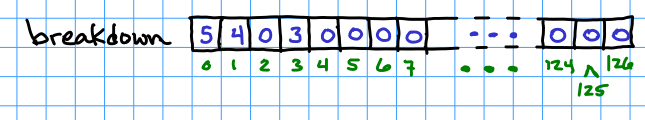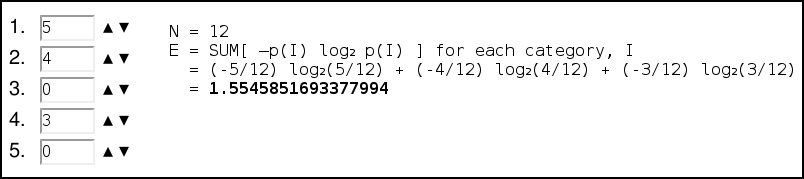# Assignment 5

Due Wednesday 21 November at 1am

The purpose of this assignment is to experiment with building decision trees using the ID3 algorithm.

# Getting started

Open any file within your `cs162` folder in the editor, and then choose the “Sync with git” option from the Tools » External Tools menu.

Now you should have an `a5` folder, with several C++ files:

``````entropy.cpp
main.cpp
Makefile
shrooms.cpp
shrooms.h
tree.cpp
tree.h``````

You are welcome to browse through all of these, but your work will mainly be in `entropy.cpp`. Open that file now, and then use Tools » External Tools » Run. You should see output like this:

``````Test: entropy 5 == 0 (PASS)
Test: entropy 4 4 == 0 (FAIL) expected 1
Test: entropy 4 3 5 == 0 (FAIL) expected 1.55459
Test: entropy 1 7 == 0 (FAIL) expected 0.54356``````

Your job will be to implement the entropy calculation, so that these tests pass. Then we will use your entropy calculation to build a decision tree to help us to decide whether to eat a (possibly poisonous) mushroom!

# Computing entropy

You should read the ID3 algorithm handout and experiment with my Entropy calculator first.

The function in `entropy.cpp` that performs the relevant calculation is this one:

``````double entropy(int breakdown[CHAR_MAX]) {
// TO DO
return 0.0;
}``````

The parameter `breakdown` is an array containing counts for up to 127 (`CHAR_MAX`) classifications. For example:The illustrated array represents that there are 5 items in category 0, 4 in category 1, none in category 2, 3 in category 3, and the rest are all zero (unused).

You can type these into the entropy calculator, and see that the result should be something like 1.5545851693377994 – we’ll round it off to 1.55459.If you look in the `main` function at the bottom of `entropy.cpp`, you also see several test cases for the entropy function:

``````    test_entropy(0.0, 5);
test_entropy(1.0, 4, 4);
test_entropy(1.55459, 4, 3, 5);
test_entropy(0.54356, 1, 7);``````

The third one covers this case: the expected value (1.55459) is provided as the first parameter, and the remaining parameters are the numbers in each category (the ordering of categories doesn’t matter when we’re doing these calculations).

To do the calculation, you’ll first need a variable `count` to reflect, the total number of items across all categories. Use a loop to add up the breakdowns. In the example, you should get 5+4+0+3 (plus a bunch more zeroes), which is 12.

Next, you need to do the log calculations. You can use a C function `log2` to compute the base-2 logarithm. For each non-zero termin the breakdown array, you’ll compute. The sum of these terms is the entropy.

Get your function to work with all the test cases given, then use the entropy calculator and add three new test cases. They should all pass.

# Shrooms!

Now, uncomment the following line in `main`:

``    shroom_main();``

This will enable the ID3 algorithm to build a decision tree, using your entropy computation. The data we will use are located in `shrooms.cpp`. The first part of this file defines the attributes of mushrooms, such as cap shape and odor:

``````const char* mushroomAttributes[NUM_ATTRIBUTES] = {
"classification",           // edible=e, poisonous=p
"cap-shape",                // bell=b,conical=c,convex=x,flat=f,
// knobbed=k,sunken=s
"cap-surface",              // fibrous=f,grooves=g,scaly=y,smooth=s
"cap-color",                // brown=n,buff=b,cinnamon=c,gray=g,green=r,
// pink=p,purple=u,red=e,white=w,yellow=y
"bruises?",                 // bruises=t,no=f
"odor",                     // almond=a,anise=l,creosote=c,fishy=y,foul=f,
// musty=m,none=n,pungent=p,spicy=s``````

We use single characters to represent each possible value, for example, `cap-color == r` means that the color of the cap is green.

Below the attribute definitions is the complete mushroom data. They begin like this:

``````char mushroomData [NUM_SAMPLES][NUM_ATTRIBUTES] = {
{'p','x','s','n','t','p','f','c','n','k','e','e','s','s','w','w','p','w','o','p','k','s','u'},
{'e','x','s','y','t','a','f','c','b','k','e','c','s','s','w','w','p','w','o','p','n','n','g'},``````

The classification is all the way to the left. So the first example here is poisonous, and the second example is edible. There are 8,124 examples in the database.

When you run your program now, after your entropy test cases, it will ask you what portion of the data to use for training. Enter a number between 0 and 1:

``````=========== ID3 Decision Tree Learning

What portion of data should we use for training? .8
6499 in training set, 1625 in test set.``````

In this case, we entered `.8`. The program will now randomize the examples, and look at 6,499 of them (80% of 8124) to build a decision tree. Then it will test that tree on the remaining 1,625 examples, and see how many it got right.

Part of the correct tree is given below, I’ll let your program figure out the rest.

``````   odor == a         # almond
E
odor == l      # anise
E
P``````

I added the comments to indicate the meaning of the one-letter abbreviations for the odor. The indentation here indicates the structure of the tree. If the odor is of almonds, then the mushroom is considered edible (E). Otherwise, if the odor is of anise, it’s still considered edible. Otherwise (neither almonds nor anise), it’s categorized as poisonous (P).

If your `entropy` function is working, the tree you get will be much larger. Try drawing the tree in a traditional format.

Using 80% of the data for training will usually be enough to get 100% of the remaining data correct, but experiment with other ratios and see what happens.

Commit your code and observations using “Sync with git.”